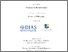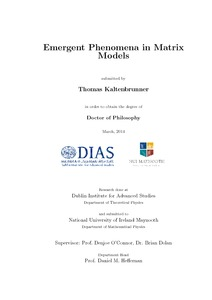# Emergent Phenomena in Matrix Models

Kaltenbrunner, Thomas (2014) Emergent Phenomena in Matrix Models. PhD thesis, National University of Ireland Maynooth.Previewmore...Add this article to your Mendeley library

## Abstract

In this work we perform a careful study of different matrix models and particularly the property of emergent phenomena in them. We start discussing a 2-matrix model of Yang-Mills type that exhibits an emergent topology in the strong coupling limit. We use Monte-Carlo simulations to obtain various observables that allow us to get more insight in the transition from the non-commutative regime towards the commutative, strong coupling, limit. We will continue to discuss higher dimensional Yang-Mills matrix models, focusing on the lowest dimensional case that is well defned, D = 3, and on the large-D limit. While we discuss the possibility of an emergent topology in 3 dimensions, we find that the behaviour of this type of models changes towards random matrices for large D. In the second part of the thesis we will add a Myers term to the Yang-Mills type models which extends the possible solutions to the model by fuzzy spaces. We carry out a 1-loop calculation for a general SU(d) symmetric solution to this class of models. We will then turn to a numerical study of a model that incorporates the simplest case of a fuzzy manifold, the fuzzy sphere. We will further study fuzzy CP2 which appears as a solution to the 8-dimensional Yang-Mills-Myers model. Numerical results from a Monte-Carlo simulation will be used to compare with the analytical results obtained earlier. We will further construct a slightly modified 8-dimensional model that has a fuzzy complex projective plane as the ground state in phase space. In total, we find four different phases in this model, which we will describe in detail after numerically mapping the phase diagram.

Item Type: Thesis (PhD) Emergent Phenomena; Matrix Models; Faculty of Science and Engineering > Mathematical Physics 5003 IR eTheses 04 Jun 2014 11:44Item control page